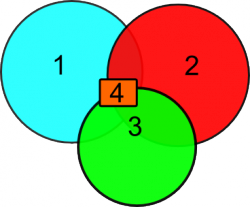# Primitives

## Overview

Primitives are the building blocks to create the scattering objects, field sources and field dumps. Each primitive has a shape (Curve, Polygon, Box, Sphere, ...) and a physical property defining its function. The physical property can be the material type (dielectric, metal, dispersive,...), a field source or a field dump to save the simulated field for post processing. All primitives for one simulation are stored in one CSXCAD struct.

List of all available primitives:

More complex structures can be created by combining various primitives. A metal sheet with cylindrical holes can be achieved by combining a metal box with several air cylinders.

Other examples of more complex structures:

## Arguments

All primitives have at least the following arguments:

• CSX: The original CSX structure
• propName: name of the assigned property
• prio: priority of the primitive (see below)

According to the shape and nature of the primitive there will be more arguments to define the size, shape, orientation and other properties which are specific to each primitive.

## Variable arguments

### Transformation

All primitives can be translated, scaled and rotated with the 'Transform' option. This option is added to the command which creates a primitive (AddBox, AddSphere, etc.), e.g.: 'Transform', {'Scale','1,1,2','Rotate_X',pi/4,'Translate','0,0,100'}. The transformations are applied in the order as given inside the {} brackets. That means, {'Scale','1,1,2','Rotate_X', pi/4} and {'Rotate_X','Scale','1,1,2'} will lead to different results.

The following transformations are available in openEMS:

• {'Scale','s'}: this will scale the primitive in x, y and z-direction equally by the factors s
• {'Scale','s_x,s_y,s_z'}: this will scale the primitive in x, y and z-direction by the factors s_x, s_y,s_z
• {'Rotate_Origin', [ax,ay,az,angle]}: this will rotate the primitive by the given angle (in radians) around the given axis [0,0,0] --> [ax,ay,az]
• {'Rotate_X', angle}: this will rotate the primitive by the given angle (in radians). Rotations around the y and z axis are done accordingly with 'Rotate_Y' and 'Rotate_Z'
• {'Translate', 't_x,t_y,t_z'}: This will translate the structure by the vector (t_x,t_y,t_z)
• {'Matrix', [4x4 matrix]}: General affine transformation given as a 4 by 4 matrix. A 1 is appended internally to the row vector of the primtive nodes, so it becomes a four-dimensional row vector. A 0 is appended to edge vectors (directions). This row vector multiplied by the transform matrix gives the new coodinates. The first three rows/columns of the matrix are rotation/shear/scaling. The last column is [0;0;0;1]. The last row is [shift_x, shift_y, shift_z, 1].

These transformations can be used to create for example an ellipsoid primitive.

### Coordinate System Definition

The coordinates used to define a primitive are always assumed to be given in the coordinate system used globally. In certain situations it may be useful though to define a primitive in a coordinate system specifically for this single primitive. The keyword for that is 'CoordSystem' and 0 for Cartesian and 1 for a cylindrical coordinate system.

## PrioritiesAt points where two or more primitives overlap, the properties of the primitive with the highest priority value will be assigned

An important concept in openEMS are the priorities assigned to each primitive. In regions where two or more primitives overlap, openEMS needs to decide which material property to assign to these regions. Here the priorities of the overlapping primitives become important: At each point openEMS will assign the material of the primitive with the highest priority. This way one can for example carve holes in a block of solid material by adding air primitives which have a higher priority. The diagrams below show how priorities will effect the material distribution for the simulations. If two blocks are overlapping, the one with the higher priority value will be chosen. For the more complicated case, where three or more blocks overlap, openEMS uses the block with the highest priority at each point. This way it is possible to create complicated shapes from the basic primitives by simply using primitives assigned to different materials and assign the priorities appropriately.

Exceptions:

• Non-physical properties, such as dump boxes, probe boxes, etc. are not affected by the priority system.
• Excitations (AddExcitation) are not affected as well, since multiple (super positioned) excitations are possible.
• The curve primitive as a 1D line element is not affected
• Lumped elements are not affected

Example:

• Define a box forcing a cylindrical coordinate representation:
```CSX = AddBox(CSX,'metal',1,[50 pi/2 10],[70 3*pi/2 -50], 'CoordSystem', 1);
```